# Circles

In the circle with a radius 7.5 cm are constructed two parallel chord whose lengths are 9 cm and 12 cm. Calculate the distance of these chords (if there are two possible solutions write both).

x =  10.5 cm
y =  1.5 cm

### Step-by-step explanation:Did you find an error or inaccuracy? Feel free to write us. Thank you!Tips to related online calculators
Do you want to convert length units?
Pythagorean theorem is the base for the right triangle calculator.

#### You need to know the following knowledge to solve this word math problem:

We encourage you to watch this tutorial video on this math problem:

## Related math problems and questions:

• Two chords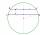In a circle with a radius of 8.5 cm, two parallel chords are constructed, the lengths of which are 9 cm and 12 cm. Find the distance of the chords in a circle.
• Two chordsIn a circle with radius r = 26 cm two parallel chords are drawn. One chord has a length t1 = 48 cm and the second has a length t2 = 20 cm, with the center lying between them. Calculate the distance of two chords.
• Two parallel chords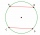The two parallel chords of the circle have the same length of 6 cm and are 8 cm apart. Calculate the radius of the circle.
• Two parallel chordsIn a circle 70 cm in diameter, two parallel chords are drawn so that the center of the circle lies between the chords. Calculate the distance of these chords if one of them is 42 cm long and the second 56 cm.
• Chors centers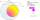The circle with a diameter 17 cm, upper chord /CD/ = 10.2 cm and bottom chord /EF/ = 7.5 cm. The midpoints of the chords H, G is that /EH/ = 1/2 /EF/ and /CG/ = 1/2 /CD/. Determine the distance between the G and H, if CD II EF (parallel).
• Touch circle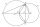Point A has a distance IA, kl = 10 cm from a circle k with radius r = 4 cm and center S. Calculate: a) the distance of point A from the point of contact T if the tangent to the circle is drawn from point A b) the distance of the contact point T from the l
• Concentric circles and chordIn a circle with a diameter d = 10 cm, a chord with a length of 6 cm is constructed. What radius have the concentric circle while touch this chord?
• Concentric circlesIn the circle with diameter, 19 cm is constructed chord 9 cm long. Calculate the radius of a concentric circle that touches this chord.
• Tangent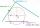What distance is the tangent t of the circle (S, 4 cm) and the chord of this circle, which is 6 cm long and parallel to the tangent t?
• Circle chordCalculate the length of the chord of the circle with radius r = 10 cm, length of which is equal to the distance from the center of the circle.
• Chord 4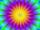I need to calculate the circumference of a circle, I know the chord length c=22 cm and the distance from the center d=29 cm chord to the circle.
• Circle's chordsIn the circle there are two chord length 30 and 34 cm. The shorter one is from the center twice than longer chord. Determine the radius of the circle.
• Two chordsCalculate the length of chord AB and perpendicular chord BC to circle if AB is 4 cm from the center of the circle and BC 8 cm from the center of the circle.
• Chord distanceThe circle k (S, 6 cm), calculate the chord distance from the center circle S when the length of the chord is t = 10 cm.
• Common chordTwo circles with radius 17 cm and 20 cm are intersect at two points. Its common chord is long 27 cm. What is the distance of the centers of these circles?
• Two chords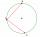There is a given circle k (center S, radius r). From point A which lies on circle k are starting two chords of length r. What angle does chords make? Draw and measure.
• ChordIn a circle with radius r=60 cm is chord 4× longer than its distance from the center. What is the length of the chord?